# McGraw Hill Math Grade 3 Chapter 5 Lesson 1 Answer Key Dividing by 2

## McGraw-Hill Math Grade 3 Answer Key Chapter 5 Lesson 1 Dividing by 2

Solve.

Divide. Use objects to help.

Question 1.
14 ÷ 2 = 7

Question 2.
6 ÷ 2 = ___Explanation:
6 ÷ 2 = 3
Six divided by two is three.

Question 3.
18 ÷ 2 = ___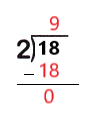Explanation:
18 ÷ 2 = 9
Eighteen divided by two is nine.

Question 4.
10 ÷ 2 = ___Explanation:
10 ÷ 2 = 5
Ten divided by two is five.

Question 5.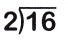Explanation:
16 ÷ 2 = 8
Sixteen divided by two is eight.

Question 6.Explanation:
2 ÷ 2 = 1
Two divided by two is one.

Question 7.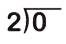Explanation:
0 ÷ 2 = 0
Zero divided by two is zero.

Question 8.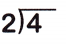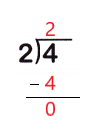Explanation:
4 ÷ 2 = 2
Four divided by two is two.

Question 9.
Tyrell has 12 cat treats. He wants to give 2 cats the same number of treats. How many treats should he give each cat?
____ ÷ ____ = ___ treats